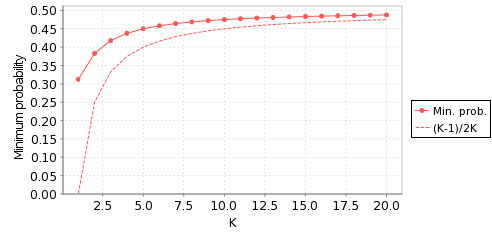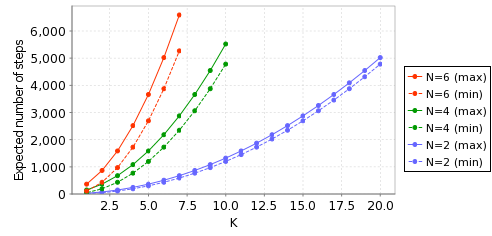# Randomised Consensus Shared Coin Protocol

(Aspnes & Herlihy)

### Contents

Related publications: [KNS01a]

### Introduction

This case study concerns modelling and verifying the shared coin protocol of the randomised consensus algorithm of Aspnes and Herlihy [AH90] using PRISM. The shared coin protocol returns a preference 1 or 2, with a certain probability, whenever requested by a process at some point in the execution of the consensus algorithm. The shared coin protocol implements a collective random walk parameterised by the number of processes N and the constant K > 1 (independent of N). The consensus algorithm proceeds through rounds and for each round r there is a new copy of the shared coin protocol denoted CFr.

The processes access a global shared counter, initially 0. On entering the protocol, a process flips a coin, and, depending on the outcome, increments or decrements the shared counter. Since we are working in a distributed scenario, several processes may simultaneously want to flip a coin, which is modelled as a nondeterministic choice between probability distributions, one for each coin flip. Having flipped the coin, the process then reads the counter, say observing c. If c >= KN it chooses 1 as its preferred value, and if c <= -KN it chooses 2. Otherwise, the process flips the coin again, and continues doing so until it observes that the counter has passed one of the barriers.

### PRISM Model

The PRISM source code in the case when there are 8 processes is given below.

```// COIN FLIPPING PROTOCOL FOR POLYNOMIAL RANDOMIZED CONSENSUS [AH90]
// gxn/dxp 20/11/00

mdp

// constants
const int N=8;
const int K;
const int range = 2*(K+1)*N;
const int counter_init = (K+1)*N;
const int left = N;
const int right = 2*(K+1)*N - N;

// shared coin
global counter : [0..range] init counter_init;

module process1

// program counter
pc1 : [0..3];
// 0 - flip
// 1 - write
// 2 - check
// 3 - finished

// local coin
coin1 : [0..1];

// flip coin
[] (pc1=0)  -> 0.5 : (coin1'=0) & (pc1'=1) + 0.5 : (coin1'=1) & (pc1'=1);
// write tails -1  (reset coin to add regularity)
[] (pc1=1) & (coin1=0) & (counter>0) -> (counter'=counter-1) & (pc1'=2) & (coin1'=0);
[] (pc1=1) & (coin1=1) & (counter<range) -> (counter'=counter+1) & (pc1'=2) & (coin1'=0);
// check
// decide tails
[] (pc1=2) & (counter<=left) -> (pc1'=3) & (coin1'=0);
[] (pc1=2) & (counter>=right) -> (pc1'=3) & (coin1'=1);
// flip again
[] (pc1=2) & (counter>left) & (counter<right) -> (pc1'=0);
// loop (all loop together when done)
[done] (pc1=3) -> (pc1'=3);

endmodule

// construct remaining processes through renaming
module process2 = process1[pc1=pc2,coin1=coin2] endmodule
module process3 = process1[pc1=pc3,coin1=coin3] endmodule
module process4 = process1[pc1=pc4,coin1=coin4] endmodule
module process5 = process1[pc1=pc5,coin1=coin5] endmodule
module process6 = process1[pc1=pc6,coin1=coin6] endmodule
module process7 = process1[pc1=pc7,coin1=coin7] endmodule
module process8 = process1[pc1=pc8,coin1=coin8] endmodule

// labels
label "finished" = pc1=3 & pc2=3 & pc3=3 & pc4=3 & pc5=3 & pc6=3 & pc7=3 & pc8=3 ;
label "all_coins_equal_0" = coin1=0 & coin2=0 & coin3=0 & coin4=0 & coin5=0 & coin6=0 & coin7=0 & coin8=0 ;
label "all_coins_equal_1" = coin1=1 & coin2=1 & coin3=1 & coin4=1 & coin5=1 & coin6=1 & coin7=1 & coin8=1 ;
label "agree" = coin1=coin2 & coin2=coin3 & coin3=coin4 & coin4=coin5 & coin5=coin6 & coin6=coin7 & coin7=coin8 ;

// rewards
rewards "steps"
true : 1;
endrewards

```

The table below shows the size of the model (number of state) for various values of N and K.

 Model: States: N K 2 2 272 4 528 8 1,040 16 2,064 32 4,112 64 8,208 4 2 22,656 4 43,136 8 84,096 16 166,016 32 329,856 6 2 1,258,240 4 2,376,448 8 4,612,864 16 9,085,696 8 2 61,018,112 4 114,757,632 8 222,236,672 16 437,194,752 10 2 2,761,248,768 4 5,179,854,848 6 7,598,460,928

### Model checking

There are two properties of the shared coin protocol we need to verify to prove Probabilistic wait free termination.

• C1: For each fair execution of CFr that starts with a reachable state of CFr, with probability 1 all processes that enter CFr leave CFr.
• C2: For each fair execution of CFr, and each value v=1,2, the probability that all processes that enter CFr will eventually leave with value v is at least (K-1)/2K.

In the case of C2, we actually calculate the exact (minimum) probability of the processes drawing the same value, as opposed to a lower bound (K-1)/2K established analytically in [AH90] using random walk theory.

### Property C1

• C1: For each fair execution of CFr that starts with a reachable state of CFr, with probability 1 all processes that enter CFr leave CFr.

We express this is PRISM as follows:

```P>=1 [ F "finished" ]
```

This is found to be satisfied in all states of the all the models listed in the table above.

### Property C2

• C2: For each fair execution of CFr, and each value v=1,2, the probability that all processes that enter CFr will eventually leave with value v is at least (K-1)/2K.

In this case, we calculate the actual minimum probability, p, for which this condition holds.

For v=1, we express this is PRISM as follows:

```Pmin=? [ F "finished"&"all_coins_equal_1" ]
```

The table below shows the computed minimum value of p and the lower bound (K-1)/2K, established analytically in [AH90] using random walk theory.

 Model: p: (K-1)/2K: N K 2 2 0.382814 0.25 4 0.437752 0.3125 8 0.468783 0.4375 16 0.484507 0.46875 32 0.492715 0.484375 64 0.498193 0.492188 4 2 0.317360 0.25 4 0.406307 0.3125 8 0.453255 0.4375 16 0.477088 0.46875 32 0.490379 0.484375 6 2 0.294365 0.25 4 0.395906 0.3125 8 0.448209 0.4375 16 0.475138 0.46875 8 2 0.282790 0.25 4 0.390749 0.3125 8 0.445831 0.4375 16 0.474747 0.46875 10 2 0.275919 0.25 4 0.387693 0.3125 6 0.425450 0.416667

For the case N=2, the following plot illustrates the results graphically:### Expected time

Finally, we compute the minimum and maximum expected number of steps required for completion of the algorithm.

We express this is PRISM as follows:

```R{"steps"}min=? [ F "finished" ]
R{"steps"}max=? [ F "finished" ]
```